# Wavevector¶

The module constructs a set of wavevectorscompatible with the reciprocal space of the periodic simulation box and grouped into shells according to their wavenumber,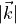. The wavevectors are of the form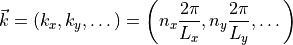where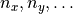are integers anddenote the edge length of the cuboid box.

Two modes are supported:

1. a sparse sampling of thin shells according to a predefined set of wavenumbers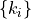, allowing for a relative deviation of the wavenumber. The number of wavevectors per shell may be limited to avoid excessively large shells for large wavenumber.
2. a dense grid of wavevectors from all points of the reciprocal lattice up to the maximum wavenumber given,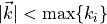. The result is grouped into shells according to the wavenumbers given. In this case, shells are half-open sets,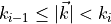, provided that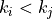for all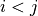.

The list of wavenumbers may be constructed using halmd.observables.utility.semilog_grid, where the smallest wavenumber is given by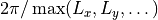.

Example:

local numeric = halmd.numeric
local utility = halmd.observables.utility

local box = halmd.mdsim.box({length={5,10,20}})

local qmin = 2 * math.pi / numeric.max(box.length)
local grid = utility.semilog_grid({start=qmin, stop=5 * math.pi, decimation=10})
local wavevector = utility.wavevector({box = box, wavenumber = grid.value, tolerance=0.05, max_count=7})

class halmd.observables.utility.wavevector(args)

Construct instance of wavevector module.

Parameters: args (table) – keyword arguments args.box – instance of halmd.mdsim.box args.wavenumber (table) – list of wavenumbers args.dense (boolean) – dense grid of wavevectors (default: false) args.tolerance (number) – relative tolerance on wavevector magnitude args.max_count (integer) – maximum number of wavevectors per wavenumber shell args.filter (table) – filter on wavevectors (default: {1, …, 1})

If dense is true, a dense grid of wavevectors is created. Otherwise, the arguments tolerance and max_count are required and a sparse sampling of wavevectors is returned.

The argument filter contains 0 or 1 for each Cartesian coordinate, 0 deletes the respective wavevector component.

wavenumber()

Returns data slot that yiels the wavenumber grid.

value()

Returns data slot that yiels the list of wavevectors grouped by their magnitude in ascending order.

__eq(other)
Parameters: other – instance of halmd.observables.utility.wavevector

Implements the equality operator a = b and returns true if the other wavevector instance is the same as this one.

halmd.observables.utility.wavevector.add_options(parser, defaults)

Add module options to command line parser: wavenumbers, tolerance, max-count.

Parameters: parser – instance of halmd.utility.program_options.argument_parser defaults (dictionary) – default values for the options